# Reason + multiplication principle - math problems

#### Number of problems found: 88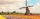How large must the group of people be so that the probability that two people have a birthday on the same day of the year is greater than 90%?
• Gold, silver, bronzeHow many ways can we divide gold, silver, bronze medals if 6 people are competing?
• Five letters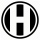How many ways can five letters be arranged?
• The testThe test contains four questions, and there are five different answers to each of them, of which only one is correct, the others are incorrect. What is the probability that a student who does not know the answer to any question will guess the right answer
• Fall sum or sameFind the probability that if you roll two dice, it will fall the sum of 10, or the same number will fall on both dice.
• Birth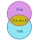Let's assume that the probability of the birth of a boy and a girl in the family is the same. What is the probability that in a family with five children, the youngest and oldest child is a boy?
• DivideHow many different ways can three people divide 7 pears and 5 apples?
• Pairs of socks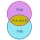Ferdinand has twelve pairs of socks, and one sock is leaky. What is the probability of putting on a leaky sock?
• Twenty-fiveHow many are three-digit natural numbers divisible by 25?
• Boys and girlsThere are 20 boys and 10 girls in the class. How many different dance pairs can we make of them?
• Two groupsThe group of 10 girls should be divided into two groups with at least 4 girls in each group. How many ways can this be done?There are 15 boys and 12 girls at the graduation party. Determine how many four couples can be selected.
• One threeWe throw two dice. What is the probability that max one three falls?
• Holidays with grandmam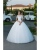We have packed three T-shirts - white, red, orange and five pairs of pants - blue, green, black, pink and yellow. How many days can we spend with the old mother if we put on a different combination of clothes every day?
• Bookshelf and books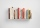How many ways can we place 7 books on a bookshelf?
• A jackpot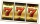How many times must I play this jackpot to win? A jackpot of seven games having (1 X 2), i. E. , home win or away win.
• Boys and girlsThere are 11 boys and 18 girls in the classroom. Three pupils will answer. What is the probability that two boys will be among them?
• Each with each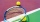Five pupils from 3A class played table tennis. How many matches did they play with each other?
• Chocolates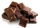How many ways can we distribute 8 different chocolates to four children?
• Three-digit numbersUse the numbers 4,5,8,9 to write all three-digit numbers without repetition. How many such numbers are there?

Do you have an exciting math question or word problem that you can't solve? Ask a question or post a math problem, and we can try to solve it.

We will send a solution to your e-mail address. Solved examples are also published here. Please enter the e-mail correctly and check whether you don't have a full mailbox.

Reason - math problems. Multiplication principle - math problems.